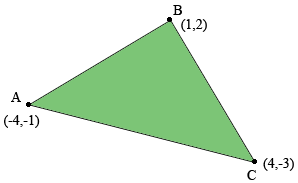SEARCH HOMEMath Central Quandaries & QueriesQuestion from Raj, a student: If the vertices of triangle ABC are A(-4,-1) B(1,2) C(4,-3) then find coordinates of circumcentre of triangleHi Raj,

The first thing you should do is draw an accurate diagram, using graph paper if at all possible. Here is my diagram.From my diagram it looks like the angle at $B$ is a right angle. Can you prove it is a right angle?

The circumcentre of a triangle is the point where the perpendicular bisectors of two sides of the triangle meet. As accurately as you can draw the perpendicular bisectors of the line segments $AB$ and $BC.$ Where does your diagram indicate they meet? Can you prove it?

PennyMath Central is supported by the University of Regina and the Imperial Oil Foundation.